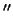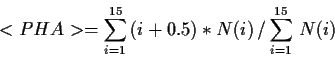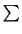Next: Quantum Efficiency Correction (a.k.a. Up: ROSAT HRI Recipes Previous: Appearance of new HRI

## Correlating Spectral Data with Spatial Gain Variations

The mean pulse height analyzer channel (PHA) of a spectral distribution will vary depending on its location on the detector. Below is a method to compare observed data with that of preflight calibration measurements. Note however that this will give only approximate values since the actual data obtained with the wobble correspond to a finite band on the detector rather than a specific location.

For temporal gain variations, see The HRI Calbration Report .

1.
Determine the roll angle of the satellite for the observation.
        cl> imhead rh*.qp | match ROLL_NOM


NOTE: The roll angle for US processed REV 0 data is given with different signs in the header compared to that of the printout (which is correct). Therefore the above method should be used only for RDF data.

2.
Retrieve the gain maps (mean PHA) from anonymous ftp at SAO. A gain map for the elements carbon (0.28 keV), copper (0.93 keV) and aluminum (1.49 keV) are available.

	> ftp sao-ftp.harvard.edu
> cd /pub/rosat/calfiles
> binary
> get gain_al.fits
> get gain_c.fits
> get gain_cu.fits
> quit


3.
Convert the gain map files to Iraf image files using the task 'dataio.rfits'

4.
Rotate one (or more) of the gain maps to the rotation of the data.
        > rotate


NOTE: use the following parameters

 rotation angle= (switch the sign of ROLL_NOM) xin=258 yin=254 xout=INDEF yout=INDEF ncols=512 nlines=512 interpo=poly3 boundary=constant constant=0.0

5.
Store the new gain map image using blink" in SaoImage. Under the item scale", click on blink" with the mouse button you would like the image stored under.
6.
Display the image file created from rarc2pros (rh*_im1.imh, 8pixels).
        > display rh*_im1.imh


7.
Flip between both images to determine the position and intensity of an HRI detection on the gain map.

NOTE: The wobble means that a general area of the detector/gain map will contribute to any give source.

8.
Procedure for calculating the mean PHA channel
(a)
run qpspec on the qpoe file while selecting the region of interest
(b)
calculate the mean of the distribution using the qpspec output with each channel being designated as (1.5,2.5,3.5,4.5....,15.5)whereis the summation from i=1 to i=15 N(i) is the number of counts in channel i.

9.
Compare between the observed and expected (calibration data) mean PHA. [But don't forget temporal gain variations!]Next: Quantum Efficiency Correction (a.k.a. Up: ROSAT HRI Recipes Previous: Appearance of new HRI
rsdc@cfa.harvard.edu
1998-06-10